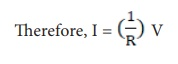Home | | Science 10th Std | Ohm‚Äôs Law

# Ohm‚Äôs Law

A German physicist, Georg Simon Ohm established the relation between the potential difference and current, which is known as Ohm‚Äôs Law. This relationship can be understood from the following activity.

OHM‚ÄôS LAW

A German physicist, Georg Simon Ohm established the relation between the potential difference and current, which is known as Ohm‚Äôs Law. This relationship can be understood from the following activity.According to Ohm‚Äôs law, at a constant temperature, the steady current ‚ÄòI‚Äô flowing through a conductor is directly proportional to the potential difference ‚ÄòV‚Äô between the two ends of the conductor.

I ‚àù V. Hence, 1/V = constant.

The value of this proportionality constant is found to be 1/RV = I R       (4.3)

Here, R is a constant for a given material (say Nichrome) at a given temperature and is known as the resistance of the material. Since, the potential difference V is proportional to the current I, the graph between V and I is a straight line for a conductor, as shown in the Figure 4.5.Study Material, Lecturing Notes, Assignment, Reference, Wiki description explanation, brief detail
10th Science : Chapter 4 : Electricity : Ohm‚Äôs Law |